# Seaborn – Sort Bars in Barplot

• Difficulty Level : Easy
• Last Updated : 09 Dec, 2021

Prerequisite: Seaborn, Barplot

In this article, we are going to see how to sort the bar in barplot using Seaborn in python.

Seaborn is an amazing visualization library for statistical graphics plotting in Python. It provides beautiful default styles and color palettes to make statistical plots more attractive. It is built on the top of matplotlib library and also closely integrated to the data structures from pandas.

### Approach:

• Import module.
• Create a Dataframe.
• Create a barplot.
• Sort the Dataframe column with DataFrame.sort_values().
• Display the sort Dataframe into the barplot.

### So, Let’s implement to sort bar in barplot using seaborn with steps based on the above approach.

Step 1: Import required packages.

## Python3

 `# Import module``import` `pandas as pd``import` `numpy as np``import` `matplotlib.pyplot as plt``import` `seaborn as sns`

Step 2: Create a Dataframe to create a barplot

## Python3

 `# Initialize data``State ``=` `[``"Rajasthan"``, ``"Bihar"``, ``"Madhya Pradesh"``,``         ``"Gujarat"``, ``"Maharashtra"``]``growth ``=` `[``342239``, ``94163``, ``308245``, ``196024``, ``307713``]` `# Create a pandas dataframe``df ``=` `pd.DataFrame({``"State"``: State,``                   ``"Growth"``: growth})` `# Display Dataframe``df`

Output: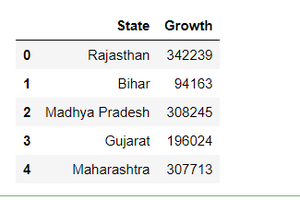Step 3: Create a barplot with this Dataframe.

## Python3

 `# make barplot``sns.barplot(x``=``'State'``, y``=``"Growth"``, data``=``df)`

Output: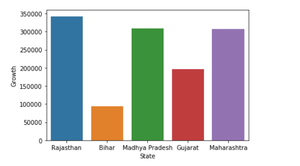Step 4: Let’s sort the Dataframe column(Growth column) with DataFrame.sort_values().

## Python3

 `# sort dataframe``df.sort_values(``'Growth'``)`

Output: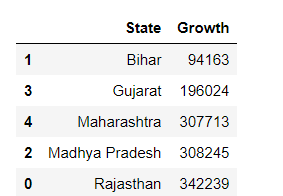We can do this same technique in a barplot.

## Python3

 `# make barplot and sort bars``sns.barplot(x``=``'State'``,``            ``y``=``"Growth"``, data``=``df,``            ``order``=``df.sort_values(``'Growth'``).State)`

Output: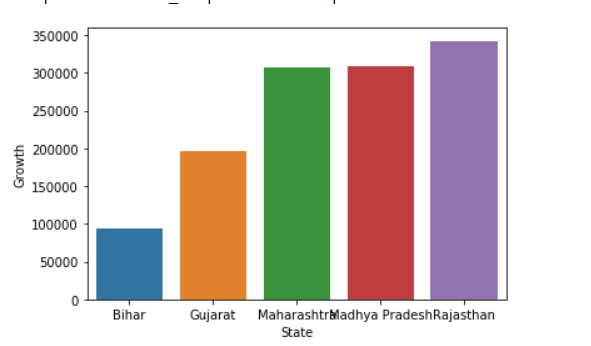Step 5: Let sort the bar with Descending order.

## Python3

 `# make barplot and sort bars``sns.barplot(x``=``'State'``,``            ``y``=``"Growth"``, data``=``df,``            ``order``=``df.sort_values(``'Growth'``,ascending ``=` `False``).State)`

Output: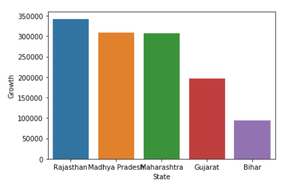Note: Default value of ascending is always True, if we change this parameter with False then it means that its arrange in descending order.

Below are the complete examples:

Example 1

## Python3

 `# import module``import` `pandas as pd``import` `numpy as np``import` `matplotlib.pyplot as plt``import` `seaborn as sns`  `# Initialize data``State ``=` `[``"Rajasthan"``, ``"Bihar"``, ``"Madhya Pradesh"``,``         ``"Gujarat"``, ``"Maharashtra"``]``growth ``=` `[``342239``, ``94163``, ``308245``, ``196024``, ``307713``]`  `# Create a pandas dataframe``df ``=` `pd.DataFrame({``"State"``: State,``                   ``"Growth"``: growth})`  `# sort dataframe``df.sort_values(``'Growth'``)`  `# make barplot and sort bars``sns.barplot(x``=``'State'``,``            ``y``=``"Growth"``, data``=``df,``            ``order``=``df.sort_values(``'Growth'``).State)`

Output:Example 2

## Python3

 `# import module``import` `pandas as pd``import` `numpy as np``import` `matplotlib.pyplot as plt``import` `seaborn as sns`  `# Initialize data``State ``=` `[``"Rajasthan"``, ``"Bihar"``, ``"Madhya Pradesh"``,``         ``"Gujarat"``, ``"Maharashtra"``]``growth ``=` `[``342239``, ``94163``, ``308245``, ``196024``, ``307713``]`  `# Create a pandas dataframe``df ``=` `pd.DataFrame({``"State"``: State,``                   ``"Growth"``: growth})`  `# sort dataframe``df.sort_values(``'Growth'``)`  `# make barplot and sort bars``sns.barplot(x``=``'State'``,``            ``y``=``"Growth"``, data``=``df,``            ``order``=``df.sort_values(``'Growth'``,ascending ``=` `False``).State)`

Output:My Personal Notes arrow_drop_up